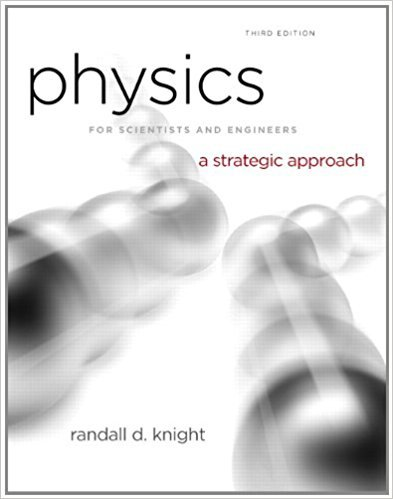×
Get Full Access to Physics For Scientists And Engineers: A Strategic Approach With Modern Physics - 3 Edition - Chapter 14 - Problem 63p
Get Full Access to Physics For Scientists And Engineers: A Strategic Approach With Modern Physics - 3 Edition - Chapter 14 - Problem 63p

×

# A molecular bond can be modeled as a spring between twoISBN: 9780321740908 69

## Solution for problem 63P Chapter 14

Physics for Scientists and Engineers: A Strategic Approach with Modern Physics | 3rd Edition

• Textbook Solutions
• 2901 Step-by-step solutions solved by professors and subject experts
• Get 24/7 help from StudySoup virtual teaching assistantsPhysics for Scientists and Engineers: A Strategic Approach with Modern Physics | 3rd Edition

4 5 1 265 Reviews
25
3
Problem 63P

Problem 63P

A molecular bond can be modeled as a spring between two atoms that vibrate with simple harmonic motion. FIGURE shows an SHM approximation for the potential energy of an HCl molecule. For E <4 × 10− 19 J it is a good approximation to the more accurate HCl potential-energy curve that was shown in FIGURE 10.31. Because the chlorine atom is so much more massive than the hydrogen atom, it is reasonable to assume that the hydrogen atom (m = 1.67 × 10−27 kg) vibrates back and forth while the chlorine atom remains at rest. Use the graph to estimate the vibrational frequency of the HCl molecule.

FIGUREStep-by-Step Solution:

Solution 63P

Step 1 of 5

The objective here is to determine the vibrational frequency of the HCl molecule from the potential energy curve given.

Step 2 of 5

Step 3 of 5

##### ISBN: 9780321740908

The full step-by-step solution to problem: 63P from chapter: 14 was answered by , our top Physics solution expert on 08/30/17, 04:34AM. This full solution covers the following key subjects: atom, figure, HCL, hydrogen, Approximation. This expansive textbook survival guide covers 17 chapters, and 1439 solutions. Physics for Scientists and Engineers: A Strategic Approach with Modern Physics was written by and is associated to the ISBN: 9780321740908. Since the solution to 63P from 14 chapter was answered, more than 1856 students have viewed the full step-by-step answer. This textbook survival guide was created for the textbook: Physics for Scientists and Engineers: A Strategic Approach with Modern Physics, edition: 3. The answer to “A molecular bond can be modeled as a spring between two atoms that vibrate with simple harmonic motion. FIGURE shows an SHM approximation for the potential energy of an HCl molecule. For E <4 × 10? 19 J it is a good approximation to the more accurate HCl potential-energy curve that was shown in FIGURE 10.31. Because the chlorine atom is so much more massive than the hydrogen atom, it is reasonable to assume that the hydrogen atom (m = 1.67 × 10?27 kg) vibrates back and forth while the chlorine atom remains at rest. Use the graph to estimate the vibrational frequency of the HCl molecule.FIGURE” is broken down into a number of easy to follow steps, and 107 words.

Unlock Textbook Solution